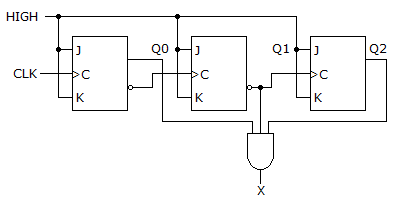# Digital Electronics - Counters

### Exercise :: Counters - General Questions

13.

When two counters are cascaded, the overall MOD number is equal to the ________ of their individual MOD numbers.

 A. product B. sum C. log D. reciprocal

Explanation:

No answer description available for this question. Let us discuss.

14.

A MOD-12 and a MOD-10 counter are cascaded. Determine the output frequency if the input clock frequency is 60 MHz.

 A. 500 kHz B. 1,500 kHz C. 6 MHz D. 5 MHz

Explanation:

No answer description available for this question. Let us discuss.

15.

Which segments of a seven-segment display would be required to be active to display the decimal digit 2?

 A. a, b, d, e, and g B. a, b, c, d, and g C. a, c, d, f, and g D. a, b, c, d, e, and f

Explanation:

No answer description available for this question. Let us discuss.

16.

How many AND gates would be required to completely decode ALL the states of a MOD-64 counter, and how many inputs must each AND gate have?

 A. 128 gates, 6 inputs to each gate B. 64 gates, 5 inputs to each gate C. 64 gates, 6 inputs to each gate D. 128 gates, 5 inputs to each gate

Explanation:

No answer description available for this question. Let us discuss.

17.

What decimal value is required to produce an output at "X" ?A. 1 B. 1 or 4 C. 2 D. 5

Explanation:

No answer description available for this question. Let us discuss.

18.

A BCD counter is a ________.

 A. binary counter B. full-modulus counter C. decade counter D. divide-by-10 counter